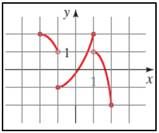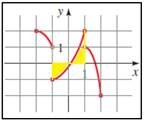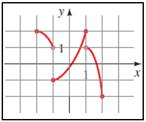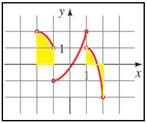# where f is increasing.### Precalculus: Mathematics for Calcu...

6th Edition
Stewart + 5 others
Publisher: Cengage Learning
ISBN: 9780840068071### Precalculus: Mathematics for Calcu...

6th Edition
Stewart + 5 others
Publisher: Cengage Learning
ISBN: 9780840068071

#### Solutions

Chapter 2.3, Problem 22E

a.

To determine

## To find: where f is increasing.

Expert Solution

f is increasing on the interval [-1,2] .

### Explanation of Solution

Given information: the graph provided in the question,From the provided information, consider,As f increases the height if the function f ( x ) also increases, thus, y-values of the points on the graph increase as the x-values increase. In the graph provided, f is increasing on the interval [-1,2]

Hence, f is increasing on the interval [-1,2] .

b.

To determine

### To find: where f is decreasing.

Expert Solution

f is decreasing on the intervals [1,2] and [1,-2].

### Explanation of Solution

Given information: the graph provided in the question,From the provided information, consider,As f decreases, the height if the function f ( x ) also decreases, thus, y-values of the points on the graph decreases as the x-values increase. In the graph provided, f is decreasing on the intervals [1,2] and [1,-2].

Hence, f is decreasing on the intervals [1,2] and [1,-2].

### Have a homework question?

Subscribe to bartleby learn! Ask subject matter experts 30 homework questions each month. Plus, you’ll have access to millions of step-by-step textbook answers!